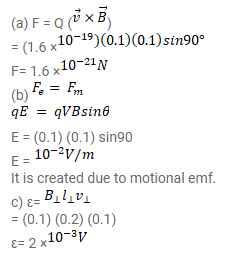# A 20 cm long conducting rod is set into pure translation with a uniform velocity of

Question:

A $20 \mathrm{~cm}$ long conducting rod is set into pure translation with a uniform velocity of $10 \mathrm{~cm} / \mathrm{s}$ perpendicular to its length. A uniform magnetic field of magnitude $0.10 \mathrm{~T}$ exists in a direction perpendicular to the plane of motion.

(a) Find the average magnetic force on the free electrons of the rod.

(b)For what electric field inside the rod, the electric force on a free electron will balance the magnetic force? How is the electric field created?

(c) Find the motional emf between the ends of the rod.

Solution: